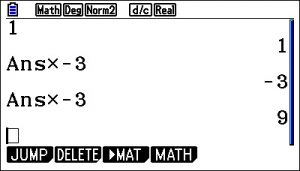# Geometric Sequences: Introduction

A geometric sequence is an ordered set, usually of numbers, where there is a common ratio between terms.

Here is an example:Here is another example:Notice that in the first example, the common ratio is 3. In the second example, the common ratio is -3.

You can create a sequence such as this on a graphical display calculator, by typing in 1, enter, multiply (-3) enter, enter, enter…# 3 kinds of common ratio

1. When the ratio is bigger than 1, the sequences gets bigger and bigger. We say it diverges.
2. When the ratio is negative, the sequence oscillates between positive and negative values.
3. When the ratio is between -1 and 1 (but not zero), the sequence diminishes. We say it converges to zero.

A geometric sequence can be defined using the first term and a common ratio. Type in your own value for first term in the box below; and your own common ratio. Slide the value ‘n’ to generate the first 20 terms and to draw a graph of your sequence on the axes. You will probably need to use the ‘move’ tool (four arrows) in the tool bar to adjust the y axis. Click on the ‘move’ tool, and put it over the y axis. Click and drag to adjust.

Here are some to try out:

• First term = 2; common ratio = 1.2 (ratio is positive, bigger than 1).
• First term = 2; common ratio = -1.2 (ratio is negative)
• First term = 2; common ratio = 0.5 (ratio is positive, less than 1)

Notice the patterns made by the points.

Did you try out a ratio value equal to zero or equal to one? What happens?

# Fill in the Gaps

The following applets generate geometric sequences. Using your own technique figure out the numbers in the gaps.

## Trickiest

I’m done! Take me back to the Sequences and Series Menu!